##### Psych 311 Unit 9 Study Guide

0
Set Details Share
created 4 years ago by wouhib
38 views
updated 4 years ago by wouhib
Page to share:
Embed this setcancel
COPY
code changes based on your size selection
Size:
X
1

Parametric tests

• Require assumptions about population parameters
• Require numerical scores
• Can be used to calculate mean & standard deviation
• Use ratio & interval data
• Testing hypotheses: Use population parameters
2

Nonparametric tests

• DO NOT require assumptions about population parameters
• DO NOT require numerical scores; use categories, names, and groupings'
• CANNOT be used to calculate mean & standard deviation
• Use nominal and ordinal data
• Testing hypotheses: DO NOT state specific, numeric, population parameters
3

When to use nonparametric tests

• When Simplicity is Needed
• Sometimes categories are simpler than scores (and still useful)
• When scores violate assumptions of parametric tests
• For instance, parametric tests require normal distributions
• When variance is extremely high
• Extreme variance makes statistical significance unlikely
• Categories allow diverse scores to fit (ex. High, Medium, Low)
• With Indeterminate or Infinite Scores
4

Research Designs for T Tests

• Single Sample
• Uses a single sample to make inferences about a single population
• Two Unrelated Samples
• Uses two samples to make inferences between two unknown populations
• Two Related Samples
• Uses one sample with each individual tested in two treatment conditions to make inferences about mean population differences
5

T Test: Hypothesis testing

• GOAL: Use sample from treated population (treated sample) to determine whether treatment has effect.
• Population mean is UNKNOWN
• Sample Mean is KNOWN, as is estimated standard error
6

T Test: Null hypothesis

• Null hypothesis states that there is no treatment effect and that the population mean is unchanged
• Null hypothesis provides a specific value for the unknown population mean
7

T Test: Reporting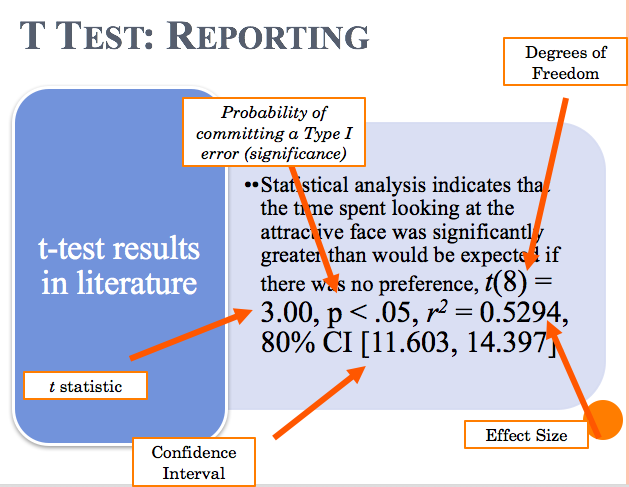8

T Test: Types

• Single Sample
• Uses sample data (one sample) to test a population mean
• Independent Samples
• Uses data from two separate samples to evaluate mean difference between two different treatment conditions or populations
• Two sets of data
• Completely separate (independent) groups of participants
• Repeated Measures Designs
• Uses two sets of data from the same sample of participants to evaluate mean difference
• Two sets of data
• The same group of participants
9

T Test: Types (reporting differences)

• Independent Samples:
• Since it involves two separate samples, extra notation is needed
• Subscripts are used, with number indicating which sample (1 or 2)
• n 1, n 2 (sample size); M 1, M 2 (means), SS 1, SS 2 (sum of squares; μ 1 - μ 2 (population mean difference)
• Repeated Measures Designs
• Uses sample mean difference (M D) to estimate population mean difference (μ D)
10

• Pro’s:
• No potential for order effects
• No potential for time-related factors
• Con’s:
• Less efficient (more participants needed)
• Less ideal for studying changes over time
• Potential problems with individual differences
11

• Pro’s:
• More efficient (fewer participants needed)
• Good for studying changes over time
• Reduces problems caused by individual differences
• Con’s:
• Potential for order effects
• Potential for time-related factors
12

T Test Vs. ANOVA

• T-test: can only compare two treatments
• ANOVA: can compare 2 or more treatments
13

ANOVA

• Definition
• Analysis of Variance
• It is a hypothesis testing procedure used to evaluate mean differences between two or more populations or treatments
• Uses sample data to make inferences about populations
• FACTOR
• The variable (independent or quasi-independent) that designates the groups being compared
• LEVELS
• Individual conditions or values that make up a factor
14

Types of ANOVA Designs

• Single-Factor
• Studies that have one independent variable (one factor)
• Independent-Measures
• Separate group of participants for each treatment being compared
• Repeated-Measures
• Same group of participants is tested in all treatment conditions
• Two-Factor
• Studies that have two independent variables (two factors)
• Can address different questions than single-factor designs
15

Null Hypothesis for ANOVA

• States that there is no treatment effect and that the population mean is unchanged
• Provides a specific value for the unknown population mean
16

Types of ALPHA

• Testwise Alpha
• The risk of a Type I error, or alpha level, for an individual hypothesis test
• This is what we’ve talked about for the whole semester
• Experimentwise Alpha
• Is the total probability of a Type I error that accumulates when several hypothesis tests are used in a single study
• Typically larger than testwise alpha
• Is the reason why multiple t-tests should be run to compare three groups (use ANOVA instead)
17

Reporting ANOVA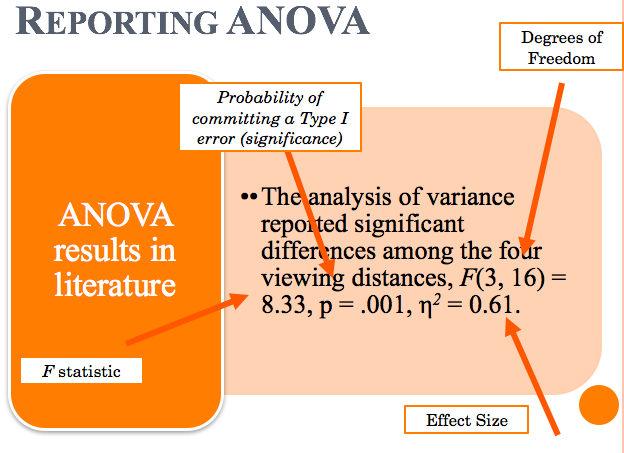18

Post-Hoc Tests

• What are they?
• Additional hypothesis tests that are run after an ANOVA to determine exactly which mean differences are significant and which are not
• When to use them?
• When you have a significant F statistic and there are more than two groups
• F-statistic does not indicate where the significance is
19

Chi-Square Test for Goodness of Fit

• Uses sample data to test hypotheses about the shape or proportion of a population distribution
• Determines how well sample proportions fit population proportions of null hypothesis
20

Chi-Square Test for Goodness of Fit: Data Presentation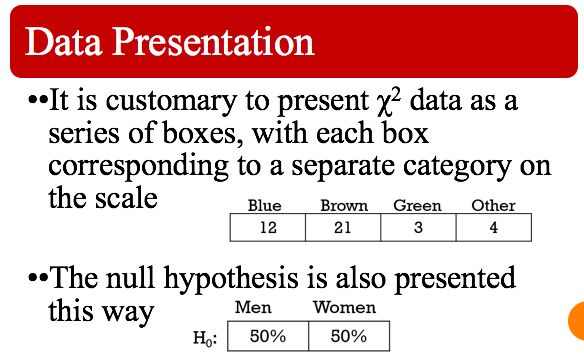21

Types of null hypotheses for Chi-Square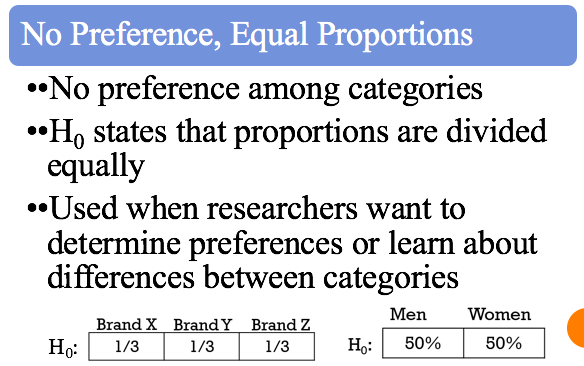22

Types of null hypotheses for Chi-Square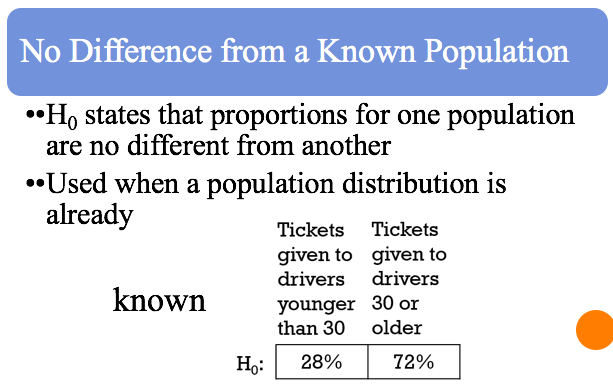23

Correlational Research Strategy

• Two or more variables are measured to obtain a set of scores (usually 2) for each individual (or source)
• Measurements are examined to identify patterns that exist between the variables and to measure the strength of the relationship
24

What correlations describe

• The direction of the relationship
• Positive or negative relationships
• The form of relationship
• Linear (using Pearson correlation)
• Monotonic (using Spearman correlation)
• The consistency or strength of relationship
• How close the relationship is to a perfect linear or a perfect monotone relationship
25

Correlational Vs. Experimental

• Correlational
• Goal: Demonstrating the existence of a relationship between variables (not explaining)
• NO manipulating or controlling variables
• Measures two different variables
• Experimental
• Goal: Demonstrating cause and effect, explaining relationship between variables
• DOES manipulate and control certain variables
• Usually only one measured (dependent) variable and looks for differences between two or more groups of scores